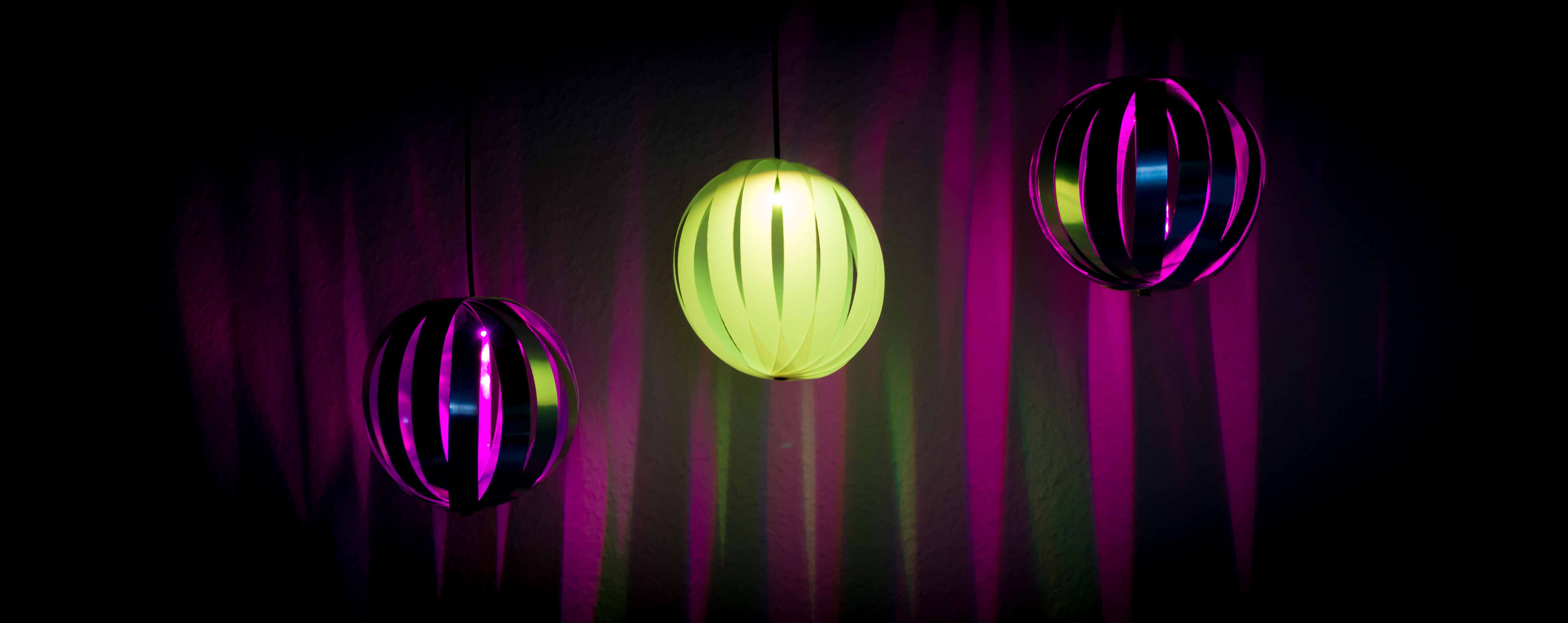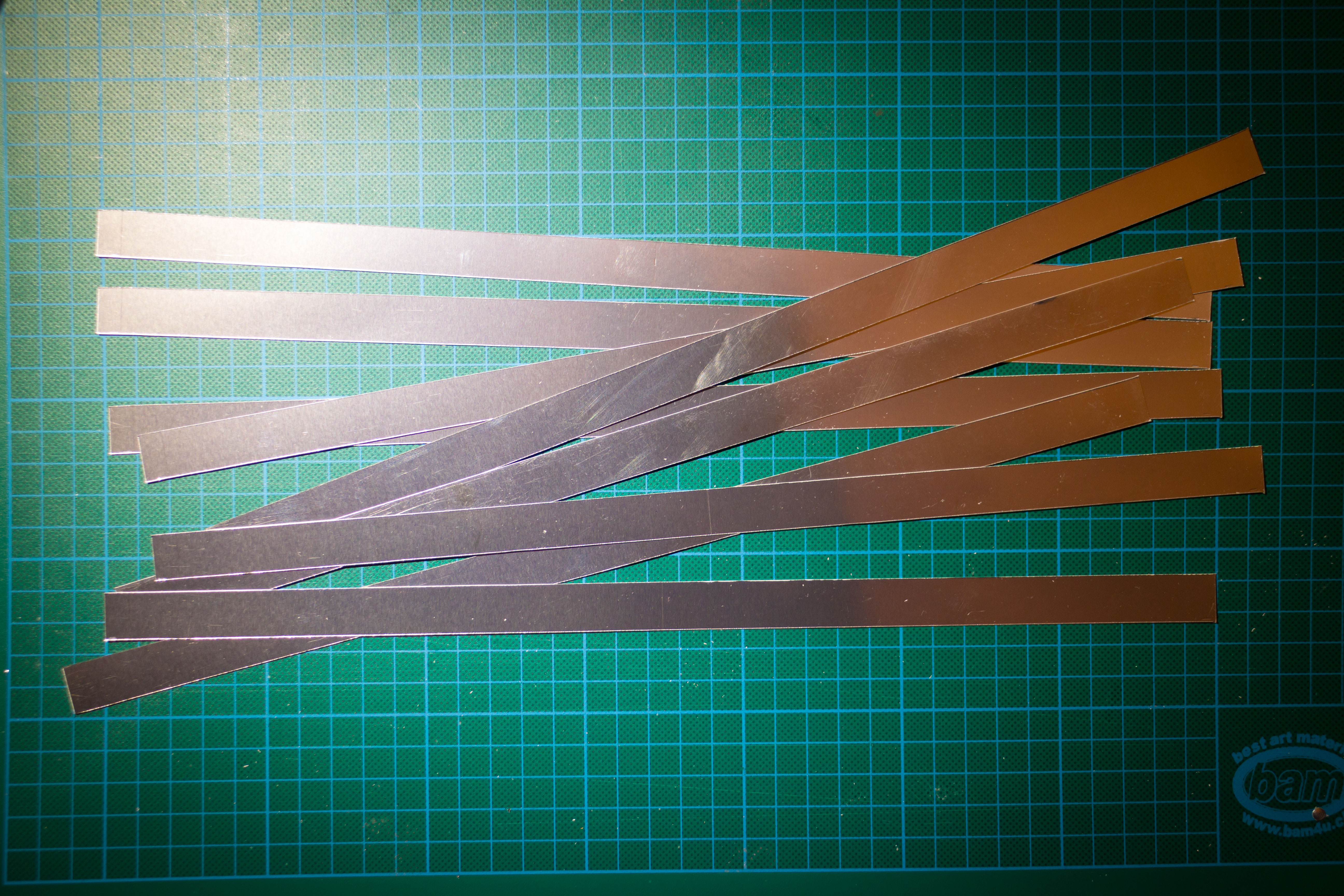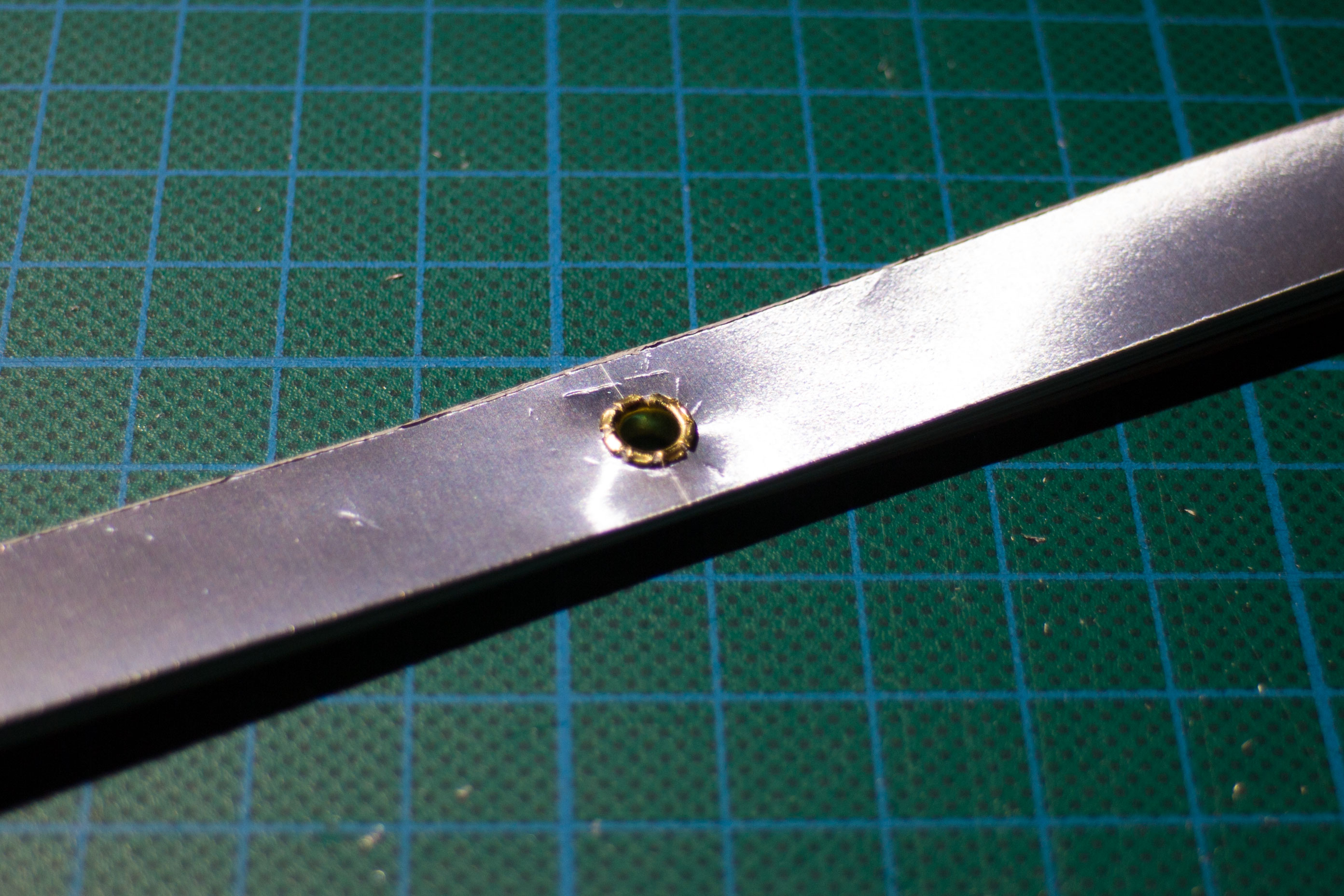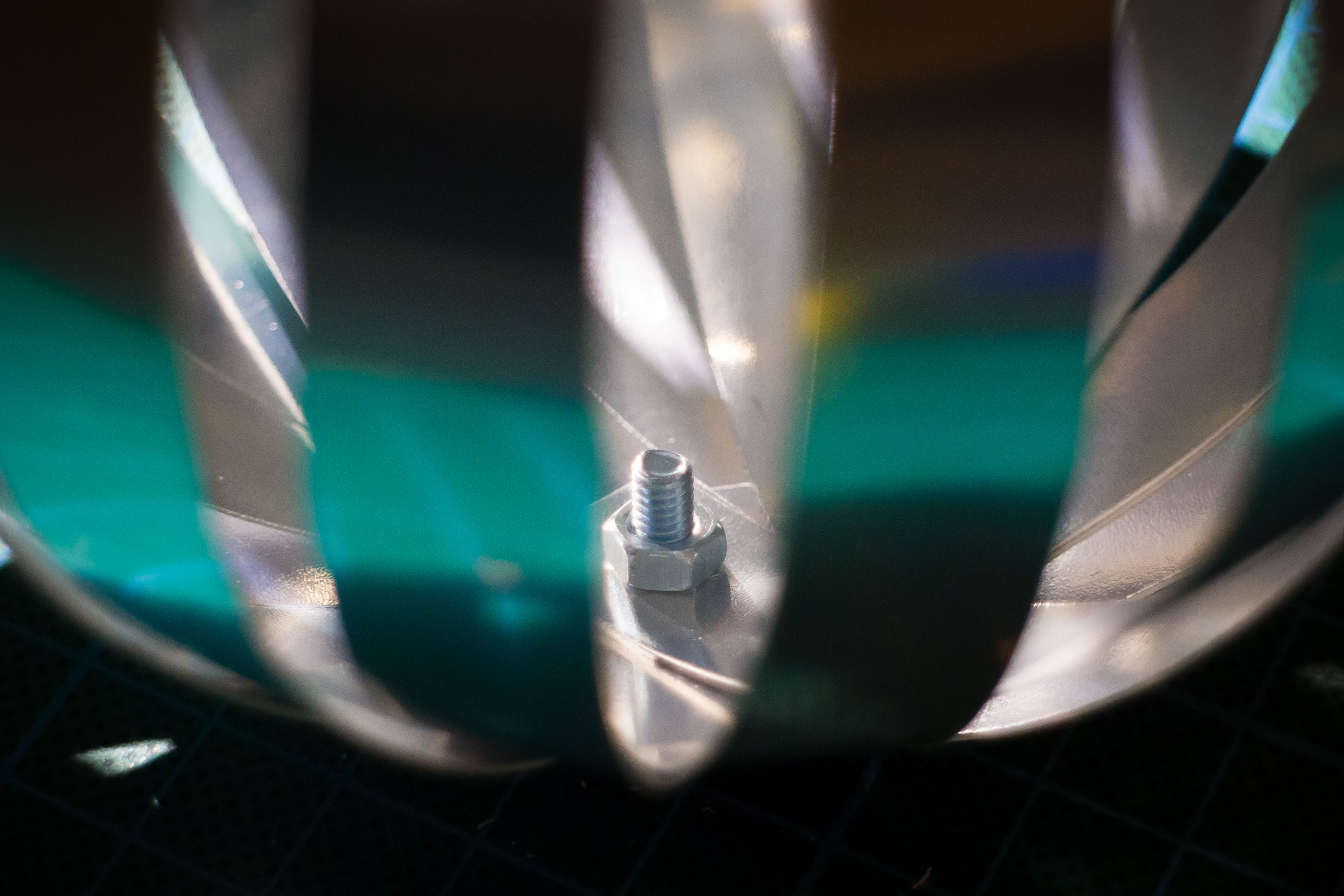# How to Create Color Changing Xmas Decoration

Update: Read the full project description here.

The days are getting shorter. You do not have to fear the dark, this is the right time to create a fancy color changing Xmas decoration. This is a very simple project and is quickly done.

## Required Parts

• “Silver” laminated paper and/or half transparent paper.
• NeoPixels from Adafruit (Flora RGB smart Neo Pixel version 2).
• Arduino Uno
• AC/DC power supply 9V, >500mA
• Thin multi conductor cable with 4 wires, or 3 wires plus shield.
• Eyelets and screws with nuts

## Create the Spheres

To create the spheres, first cut a number of stripes with equal length and width. I personally used stripes 35cm long and 1.5cm wide.Stack 8 stripes and put a hole in the middle of the stripes and two holes at the end of the stripes.Next I put an eyelet into the middle and assemble all stripes using the eyelet.Next I rotate the stripes until all are evenly distributed and have the same angle. Then I bend them up, starting with the top one and use another eyelet to connect the stripes using the holes at the sides of the stripes.In the case the eyelet is too short, I simple use a screw with a nut to fasten the stripes.## The Light

I use the wire to connect all NeoPixels. They need power and have one input and one output line. I solder the NeoPixels to the wire, connect the input and output lines.

## The COntroller

As controller I use an Arduino Uno and connect the power of the NeoPixels to the VIN and GND pins and the input of the first NeoPixel to I/O pin seven.

## Software

I first created a class `Color` for all color calculations.

```#pragma once

#include &amp;lt;Arduino.h&amp;gt;

class Color
{
public:
Color()
: _r(0.0f), _g(0.0f), _b(0.0f)
{
}

Color(float r, float g, float b)
: _r(r), _g(g), _b(b)
{
checkLimits();
}

Color(float h)  // hue
{
const int p = h*3.0f;
const float m = (h*3.0f)-((float)(p));
switch (p) {
case 0:
_r = 1.0f-m;
_g = m;
_b = 0.0f;
break;
case 1:
_r = 0.0f;
_g = 1.0f-m;
_b = m;
break;
case 2:
_r = m;
_g = 0.0f;
_b = 1.0f-m;
break;
}
checkLimits();
}

Color blendWith(const Color &amp;amp;other, float factor)
{
return Color(
((_r * (1.0f-factor)) + (other._r * factor)),
((_g * (1.0f-factor)) + (other._g * factor)),
((_b * (1.0f-factor)) + (other._b * factor)));
}

uint32_t getColor() const
{
const uint8_t r = (uint8_t)(255.0f * _r);
const uint8_t g = (uint8_t)(255.0f * _g);
const uint8_t b = (uint8_t)(255.0f * _b);
return ((uint32_t)r &amp;lt;&amp;lt; 16) | ((uint32_t)g &amp;lt;&amp;lt;  8) | b;
}

private:
void checkLimits() {
if (_r &amp;lt; 0.0f) _r = 0.0f;
if (_r &amp;gt; 1.0f) _r = 1.0f;
if (_g &amp;lt; 0.0f) _g = 0.0f;
if (_g &amp;gt; 1.0f) _g = 1.0f;
if (_b &amp;lt; 0.0f) _b = 0.0f;
if (_b &amp;gt; 1.0f) _b = 1.0f;
}

private:
float _r;
float _g;
float _b;
};
```

Then I created a very simple controller code which changes the color very smoothly every two minutes.

```#include &amp;lt;Adafruit_NeoPixel.h&amp;gt;
#include &amp;quot;Color.h&amp;quot;

void setup() {
strip.begin();
strip.show(); // Initialize all pixels to 'off'
pinMode(13, OUTPUT);
}

Color c1;
Color c2;
Color c3;

void loop() {
Color new1;
Color new2;
Color new3;
getRandomColor(&amp;amp;new1, &amp;amp;new2, &amp;amp;new3);

// blend the colors
for (float i = 0.0f; i &amp;lt; 1.0f; i += 0.001) {
strip.setPixelColor(0, c1.blendWith(new1, i).getColor());
strip.setPixelColor(1, c2.blendWith(new2, i).getColor());
strip.setPixelColor(2, c3.blendWith(new3, i).getColor());
strip.show();
delay(20);
}

// this are the new colors
c1 = new1;
c2 = new2;
c3 = new3;
strip.setPixelColor(0, c1.getColor());
strip.setPixelColor(1, c2.getColor());
strip.setPixelColor(2, c3.getColor());
strip.show();

// Show the colors for 5 minutes
for (int i = 0; i &amp;lt; 120; ++i) {
delay(1000);
digitalWrite(13, ((i&amp;amp;1)==0)?HIGH:LOW);
}
}

uint8_t getHueShift(Color *c1, Color *c2, Color *c3, float s1, float s2)
{
float h1 = (float)(random(1024))/1024.0f;
float h2 = h1 + s1;
float h3 = h1 + s2;
while (h2 &amp;gt; 1.0) h2 -= 1.0;
while (h3 &amp;gt; 1.0) h3 -= 1.0;
while (h2 &amp;lt; 0.0) h2 += 1.0;
while (h3 &amp;lt; 0.0) h3 += 1.0;
*c1 = Color(h1);
*c2 = Color(h2);
*c3 = Color(h3);
}

void getRandomColor(Color *c1, Color *c2, Color *c3)
{
uint8_t mode = random(12);
switch (mode) {
case 0:
default:
*c1 = Color(1.0, 1.0, 1.0);
*c2 = Color(0.8, 0.8, 0.8);
*c3 = Color(0.6, 0.6, 0.6);
break;
case 1:
getHueShift(c1, c2, c3, 0.0, 0.0);
break;
case 2:
getHueShift(c1, c2, c3, 0.333, 0.666);
break;
case 3:
getHueShift(c1, c2, c3, 0.5, 0.0);
break;
case 4:
getHueShift(c1, c2, c3, 0.2, 0.4);
break;
case 5:
getHueShift(c1, c2, c3, 0.1, 0.2);
break;
case 6:
getHueShift(c1, c2, c3, -0.2, -0.4);
break;
case 7:
getHueShift(c1, c2, c3, -0.1, -0.2);
break;
case 8:
getHueShift(c1, c2, c3, 0.2, 0.0);
break;
case 9:
getHueShift(c1, c2, c3, 0.4, 0.0);
break;
case 10:
getHueShift(c1, c2, c3, -0.2, 0.0);
break;
case 11:
getHueShift(c1, c2, c3, -0.4, 0.0);
break;
}
}
```

Note I am using `float` for the calculations. Usually this is a bad idea, but the controller is most of the time idle and has plenty of RAM left, so there is no big impact because of this.

This site uses Akismet to reduce spam. Learn how your comment data is processed.